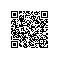# SLS机器学习介绍（05）：时间序列预测

## 一、问题背景

### 场景一：计量预测

• 我们在使用阿里云的产品，能不能提供一下我们服务未来一周或者未来一天的相关计量指标的预测，方便计算服务外来的花费等问题？

### 场景二：服务容量预测

• 能否根据历史数据，预测一下，网站请求量的情况？存储容量的预测？OSS使用情况的预测？
• 能否根据在ECS中的机器的使用情况，能否更好的辅助运维同学做各种资源的调度，让用户的服务更加弹性，提高服务的可用性？
• 能否预测稳定业务的机柜和机架的电量消耗，帮助数据中心降低资源消耗，节约成本？

### 场景三：业务预测告警

• 根据服务的历史访问情况，进行未来一天的访问请求预测，当实际值与预测值偏差较大时，立马告知相关的运营同学，分析用户留存等问题，帮助产品持续迭代？

## 二、我们提供了什么？

### 2.1 统计学模型

1. 使用默认的arma(p=3, q=1)模型进行预测
ts_predicate_simple(unixtime, val, nPred, samplePeriod, sampleMethod)
2. 使用ar(p)模型进行预测
ts_predicate_ar(unixtime, val, p, nPred, samplePeriod, sampleMethod)
3. 使用arma(p, q)模型进行预测
ts_predicate_arma(unixtime, val, p, q, nPred, samplePeriod, sampleMethod)
4. 使用arima(p, d, q)模型进行预测
ts_predicate_arima(unixtime, val, p, d, q, nPred, samplePeriod, sampleMethod)

### 2.2 机器学习模型

1. 不对数据做任何处理，直接使用GBRT模型进行预测
ts_regression_predict(unixtime, val, nPred, 'origin', samplePeriod, sampleMethod)
2. 对数据做时序分解，对分解出来的序列分别做预测，在进行整合
ts_regression_predict(unixtime, val, nPred, 'forest', samplePeriod, sampleMethod)
3. 不对数据做任何处理，使用线性模型进行预测
ts_regression_predict(unixtime, val, nPred, 'linear', samplePeriod, sampleMethod)
4. 使用auto参数，算法尽可能根据时序自身的特征，进行学习预测
ts_regression_predict(unixtime, val, nPred, 'auto', samplePeriod, sampleMethod)

## 三、内部的算法逻辑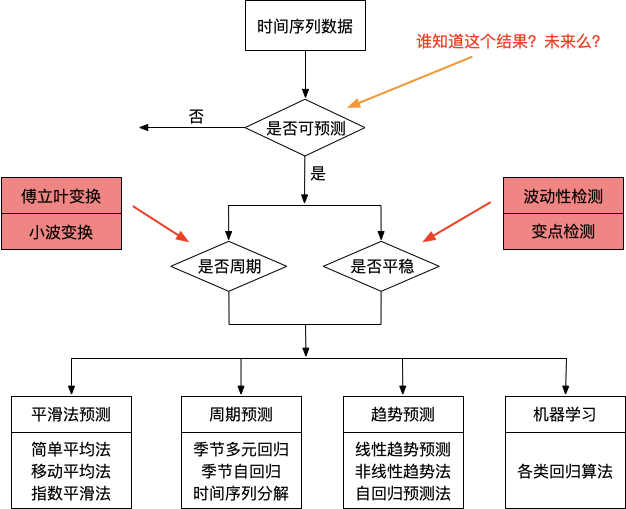### 3.0 数据的基本预处理

1. 数据先进行归一化，去均值操作，这个很重要，需要将信号中的直流分量去除让算法分析时效果更加稳定。
2. 按照一定的策略将数据进行补齐，具体的操作详见文档：Signal extension modes。这里展示出补点结果的示意图，其中红色的曲线点是原始的时序序列，其中黑色的点是按照不通方式补充的点示意图。本平台内置的算法使用的补点策略是Periodic模式。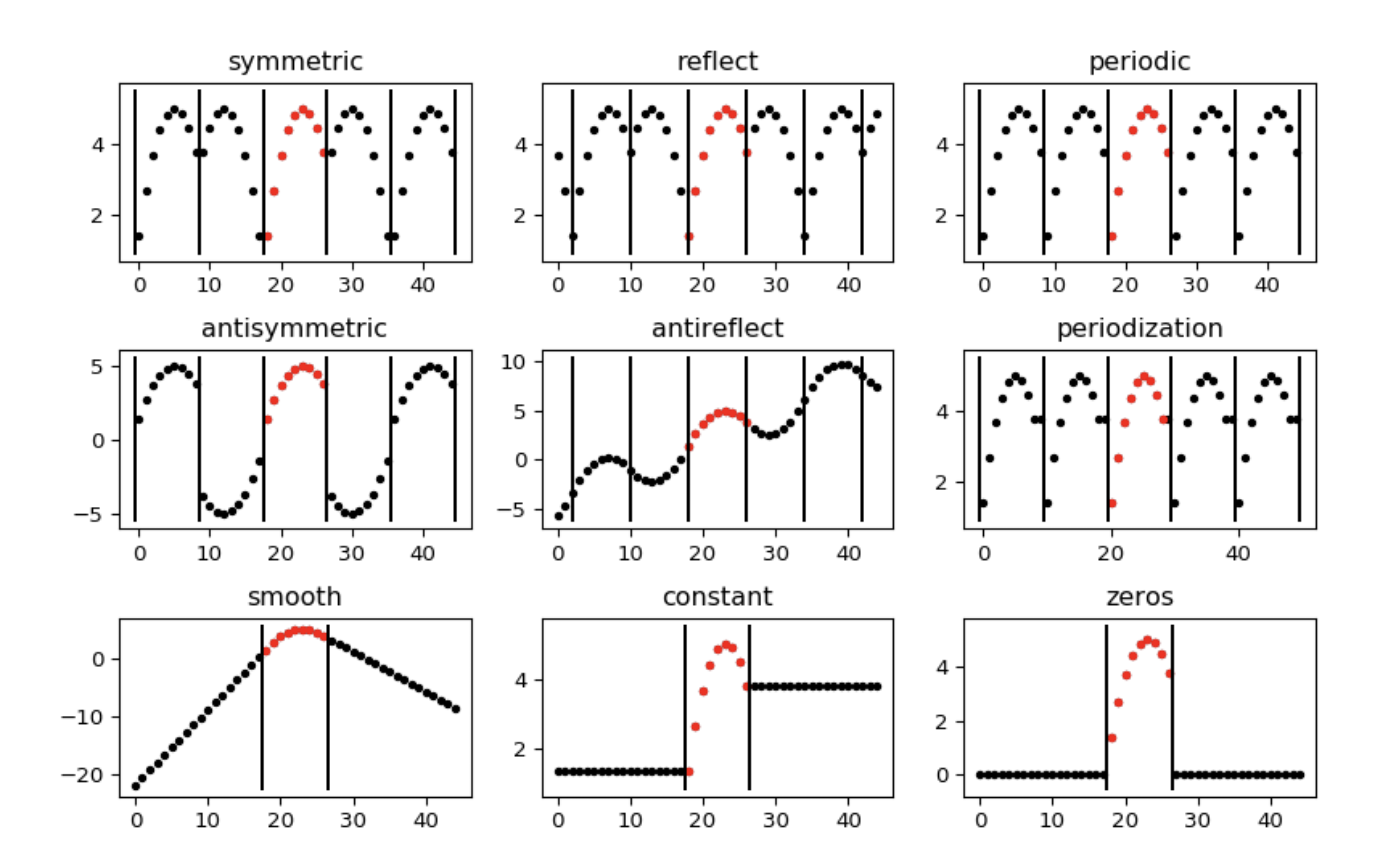### 3.1 小波分解算法

#### 3.1.1 形象的认识下小波

• 小波是啥？

• 可视化一些小波基函数

• Haar小波：Haar小波函数，是小波分析中最早使用的一个具有紧支撑的正交小波函数，也是最简单的一个小波函数。Haar小波在时域上是不连续的，所以作为基本小波性能并不是很好。
• Daubechies小波：多贝西小波，一般简写成DB-N，其中N是小波的阶数。DB-N小波具有很好的正则性，即该小波作为稀疏基所引入的光滑误差不容易被察觉，使得信号重构过程比较光滑。DB-N小波的特点是随着阶数的增加，消失矩的阶数也会越大，其中消失矩越高，重构的信号的光滑性越好，频域的局部化能力就越强，频带的划分效果越好，但是会使得支撑域减弱，同时计算量加大。
• Symlet小波：Symlet小波函数是对DB-N系列小波函数的一种改进。Symlet小波系通常表示为Sym-N。SymN小波的支撑范围为2N-1，消失矩为N，同时也具备较好的正则行。与DB-N小波相比，在连续性、支撑集长度、滤波器长度等方面与DB-N小波一致，但sym-N小波具有更好的对称性，即一定程度上能够减少对信号进行分析和重构时的相位失真。
• Meyer小波：Meyer小波的小波函数和尺度函数都是在频率域中进行定义的，它不是紧支撑的，但是它的收敛速度很快。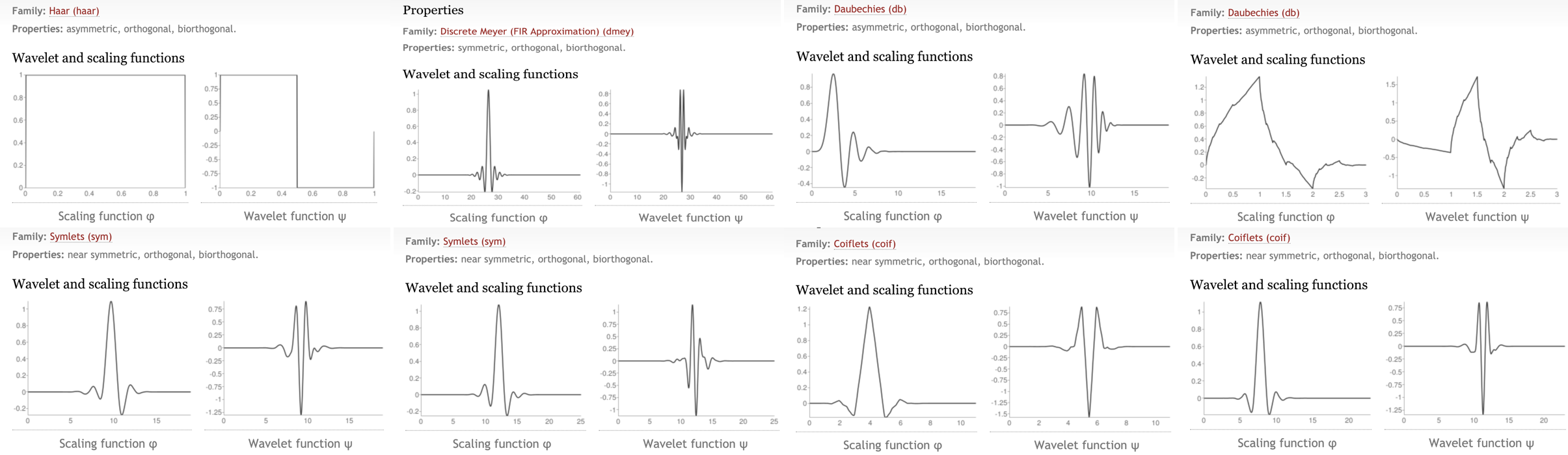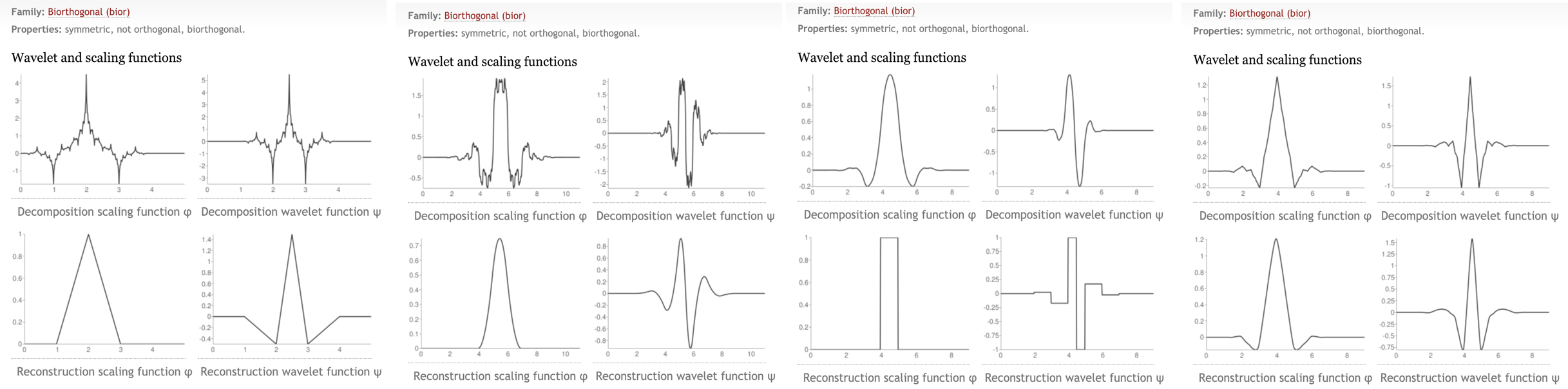#### 3.1.2 Mallat小波分解

• 序列的小波分解的具体的逻辑关系如下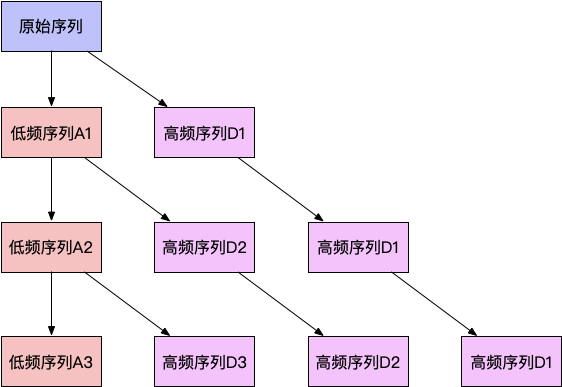• 分解逻辑解释
将时间序列进行小波分解，每一层分解的结果是上次分解得到的低频信号再分解成高频和低频两个部分，如此进行N层分解后，源信号X被分解成：

$$X = D_1 + D_2 + ... + D_N + A_N$$

1. 对源序列进行小波分解，得到各层小波系数
2. 对各层小波系数分别建立时序模型，对各层小波系数进行预测
3. 用得到的预测小波系数对原始序列进行重构

• PS：拿到各个分解出来的序列信号，还能做些什么？

• 针对低频部分的可以分别去检测序列的周期性

• 以某集群的CPU负载情况为例，来看下分析的能力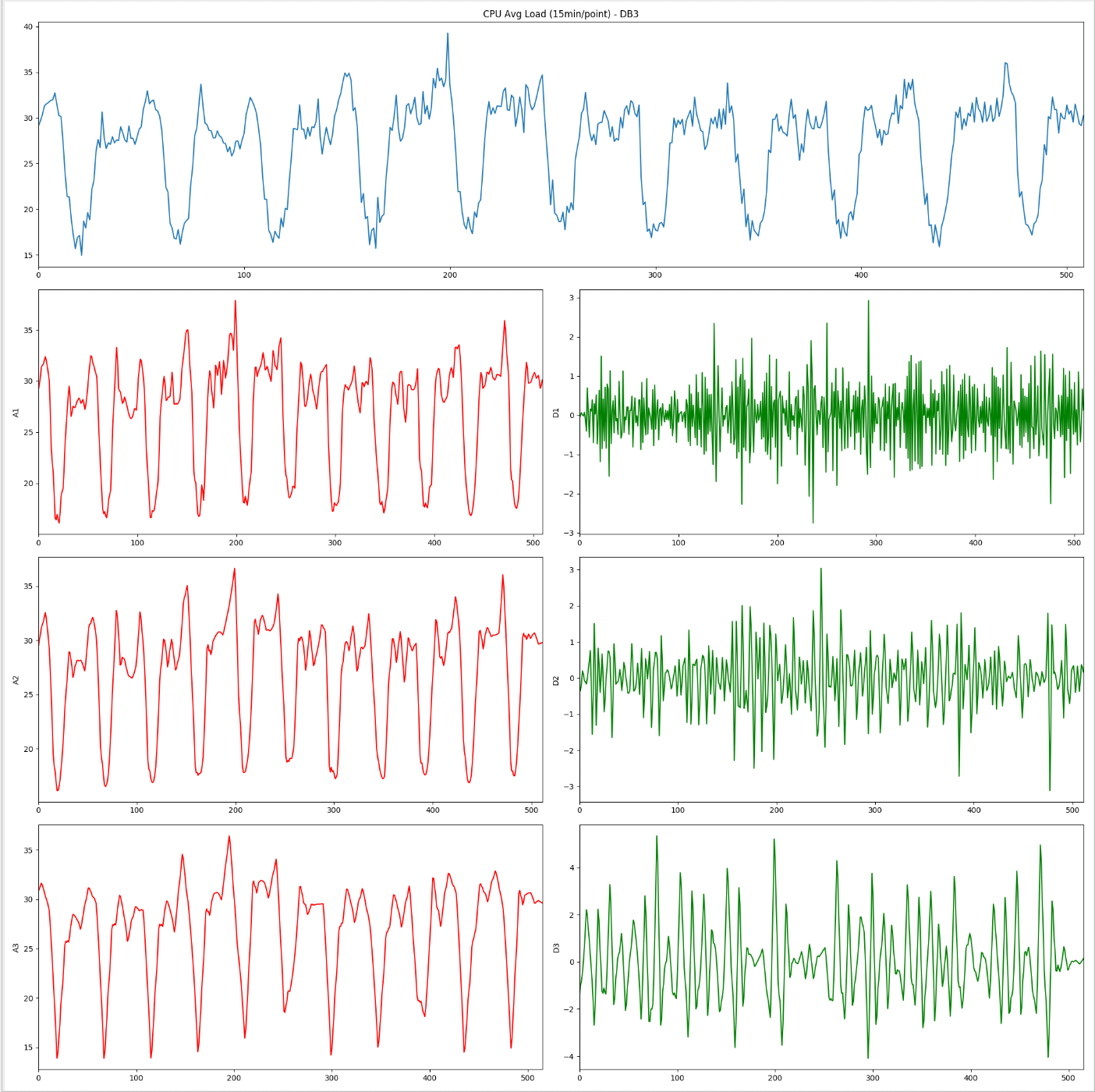• 蓝色表示15分钟一个粒度的某集群CPU负载曲线的实际图
• A1、A2、A3表示不同层级的低频信号重构出来的时域信号信息
• D1、D2、D3表示相应层级的高频信号重构出来的时域信号信息
• 某网站的近10天PV情况为例子，来看下分析能力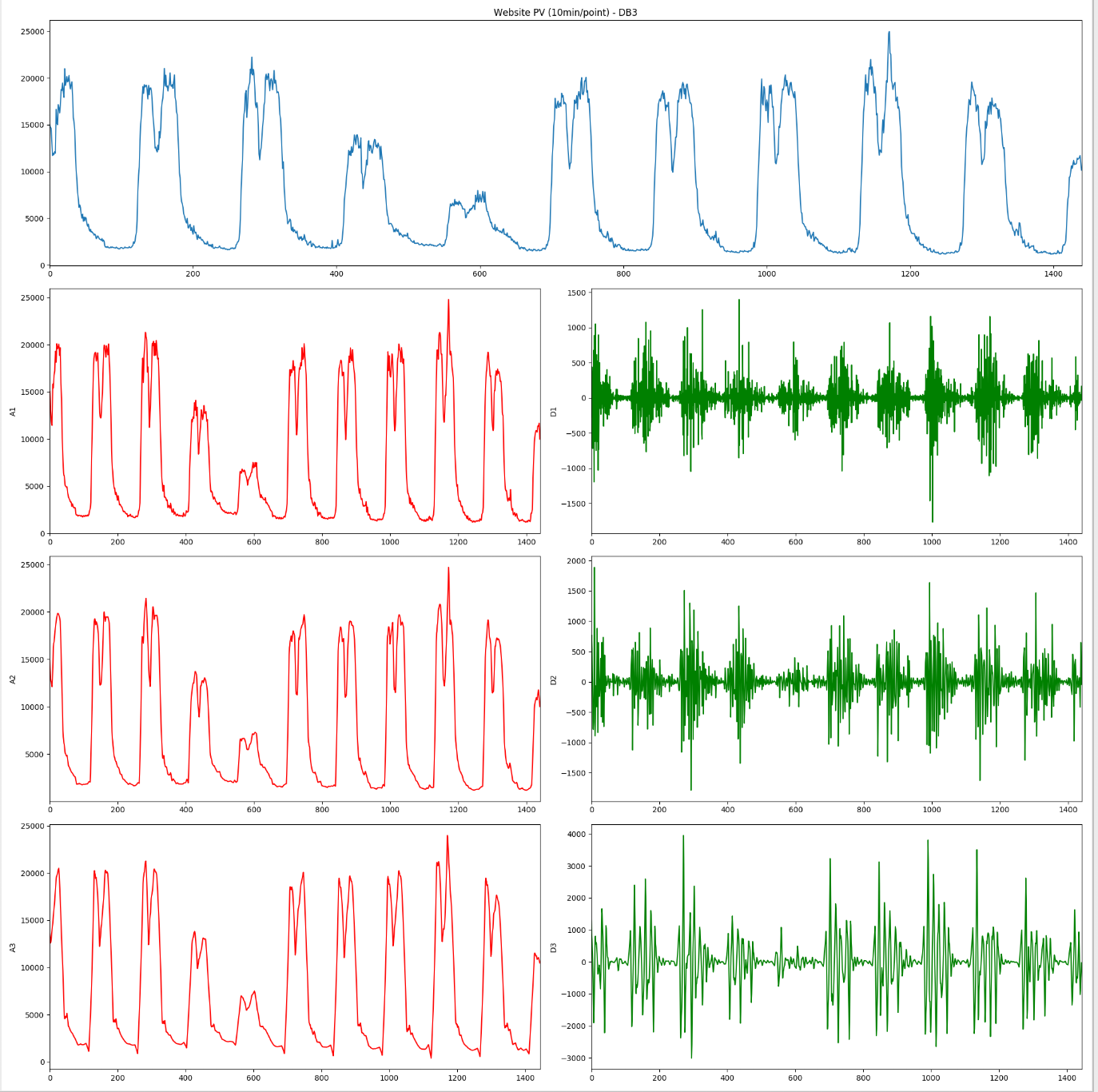• 蓝色表示10分钟一个粒度的某WebSite的PV曲线的实际图
• A1、A2、A3表示不同层级的低频信号重构出来的时域信号信息
• D1、D2、D3表示相应层级的高频信号重构出来的时域信号信息

#### 3.1.3 小波收缩(WaveletShrinkage)算法

WaveShrink方法来对未知信号进行降噪，此方法的原理是将小波系数趋向零收缩，从而达到降噪目的。一般而言，信号经小波变换后，得到一系列的小波系数。一般情况下，较大系数代表信号，较小系数代表噪声，将小系数剔除后，在进行小波逆变换，重构信号中的噪声含量便降低了。

• WaveShrink降噪分为以下三步：

• 将信号进行小波变换，求小波系数；
• 根据经验公式计算阈值，并对小波系数进行阈值处理；
• 将处理后的小波系数逆变换。阈值处理方法采用软阈值方式，软阈值方式是讲小波系数通阈值相比较，绝对值小于阈值的系数被设置成零，绝对值大于阈值的系数将阈值从其绝对值中减掉；
• 经过软阈值降噪的信号比较平滑，但是信号会产生一定的偏差；
• 基于Stein's Unbiased Risk Estimate原理（SURE）产生阈值

• 对于一个给定的阈值$\lambda$最小化，就得到了所选择的阈值，给定阈值$\lambda$定义为：

$$\lambda = \sigma * 2 * \sqrt(log(n) / n), \sigma = MAD / 0.6745$$

MAD为最佳尺度上小波系数轨迹的绝对均值，因子0.6745是高斯分布矫正选择的。

• 以某集群中某机柜的电量使用情况，可以在具体的使用的效果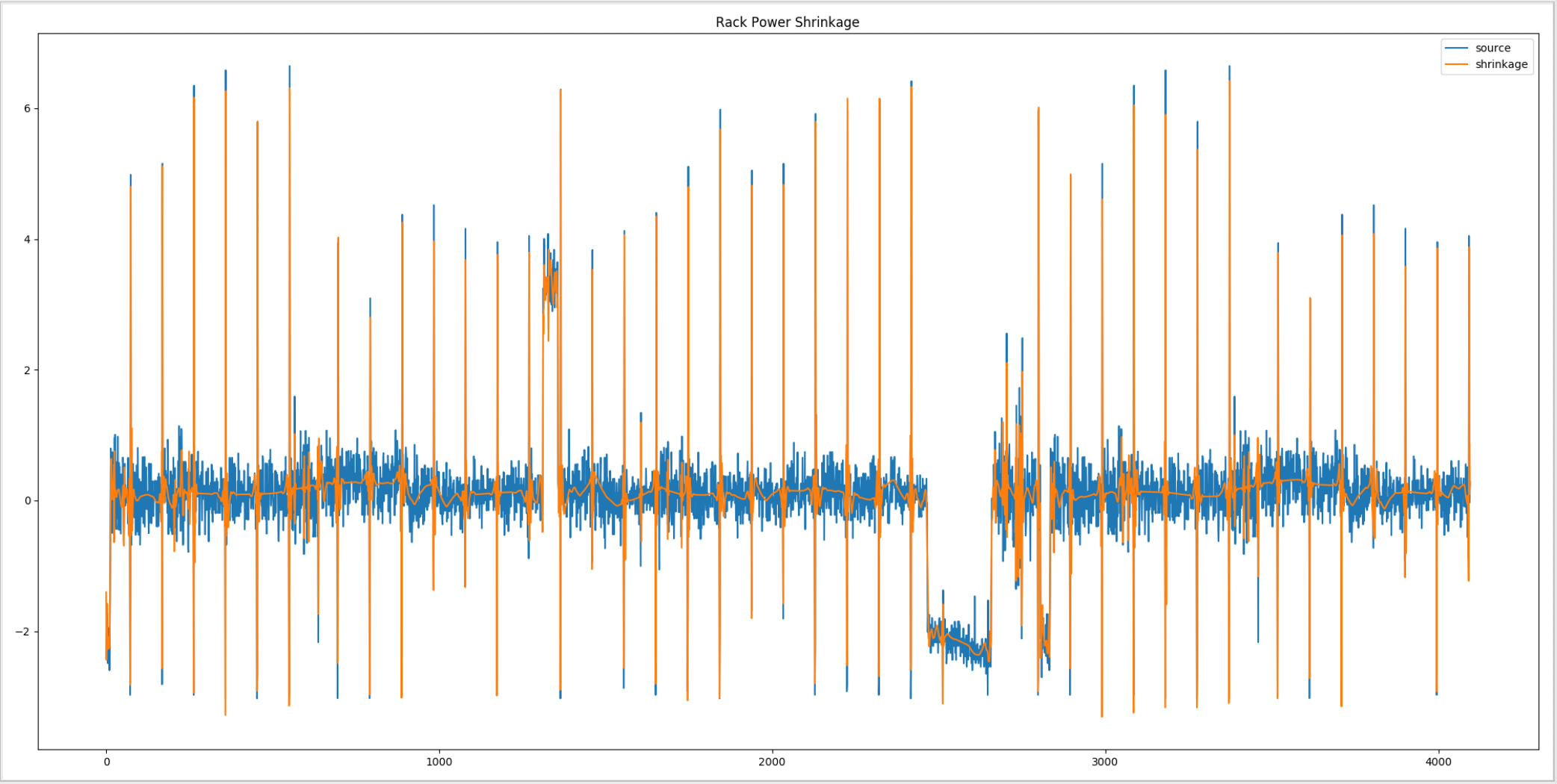### 3.2 机器学习算法

#### 3.2.0 模型构建和验证流程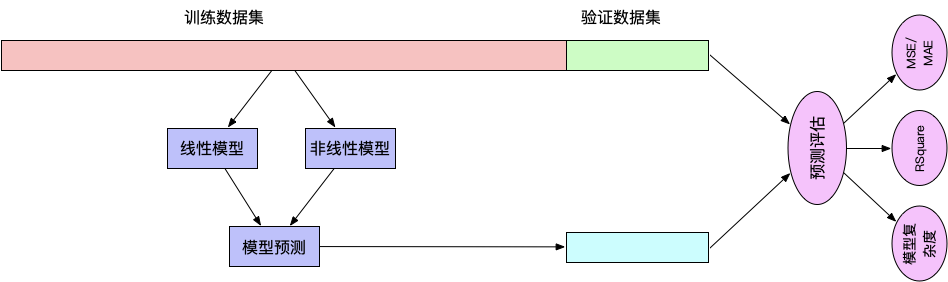• 直接比较不同模型在相同的训练集中的效果如何？或是相同模型的不同参数在同一个数据集合中的效果如何？
• 在另一份数据中去验证模型在不同指标的好坏，最终通过相关的结果评估公式选择一个目前最好的模型作为最终的模型
• 当前讨论问题是对时序进行预测，因此，模型衡量的标准就是在验证集合中时序的预测效果，衡量效果的指标就是MAE/MSE/R-Square/Adj-R-Square。

#### 3.2.1 线性模型（Linear Regression）

$$Y = F(X_1,X_2,X_3) = a*X_1 + b*X_2 + c*X_3 + d$$

• 常用的线性模型

• 线性回归
• 多元线性回归
• 岭回归
• Lasso回归

$$f(x_i) = \sum_{m=1}^{p} w_m * x_{im} + w_0 = w^{T} * x_i$$

#### 3.2.3 非线性模型（Non-Linear Regression）

• 机器学习算法可以进行回归预测的模型如下

• Logistic Regression
• GBRT（MART）/XGBOOST
• 神经网络模型
• 支持向量机（SVR）
• 不同学习方法在不同特性方面的体现

PS：上述表格出自《统计机器学习基础：数据挖掘、推理与预测》第10章。

##### 3.2.3.1 回归树算法简介

$$f(x) = \sum_{m=1}^{M}c_m * I(x \in R_m)$$

$$\hat{c}_m = avg(y_i | x_i \in R_m)$$

$$R_1(j, s) = \{X|X_j \leq s\} , R_2(j, s) = \{X | X_j > s\}$$

$$min_{j,s} = [min_{c_1} \sum_{x_i \in R_1(j, s)}(y_i - c_1)^2 + min_{c_2} \sum_{x_i \in R_2(j, s)}(y_i - c_2)^2]$$

$$\hat{c}_1 = avg(y_i|x_i \in R_1(j, s)), \hat{c}_2 = avg(y_i|x_i \in R_2(j, s))$$

PS：这仅仅是回归算法的简单描述，并没有详细说明其中越到的各种坑。

##### 3.2.3.2 时序特征的构造## 硬广时间

### 联系我们## 参考文献